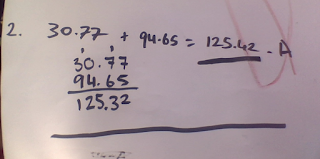Monday, July 2, 2018

DMIC - a new way in maths!

WALT: Work collaboratively to solve word problemsMy group and I were learning about decimals, in my group was : Ana, Myztique, Bryana, Uasi, and of course myself. We had to read the word problem and solve it Keep on reading to find out more!!For the first question was 4.26 + 7.54 + 3.90 = 15.7, the first thing we did was

4.26 + 7.54 = 11.8
then 11.8 + 3.90 = 15.7 so the answer is 15.7The second question was 30.77 + 94.65 = 125.32

30.77
94.65
125.32